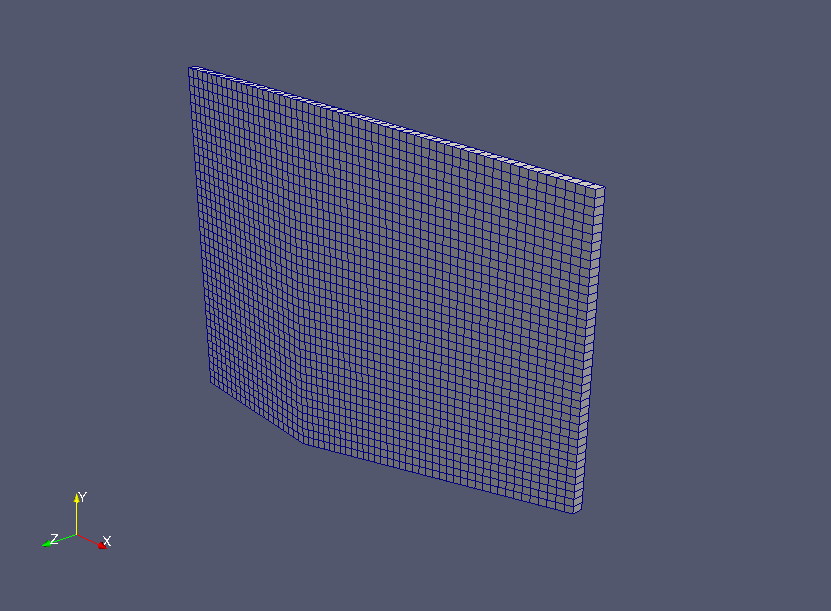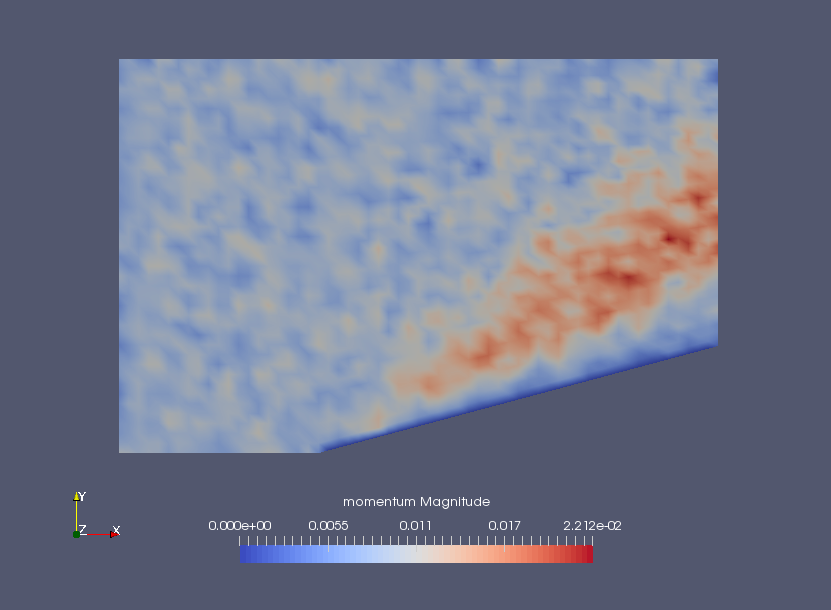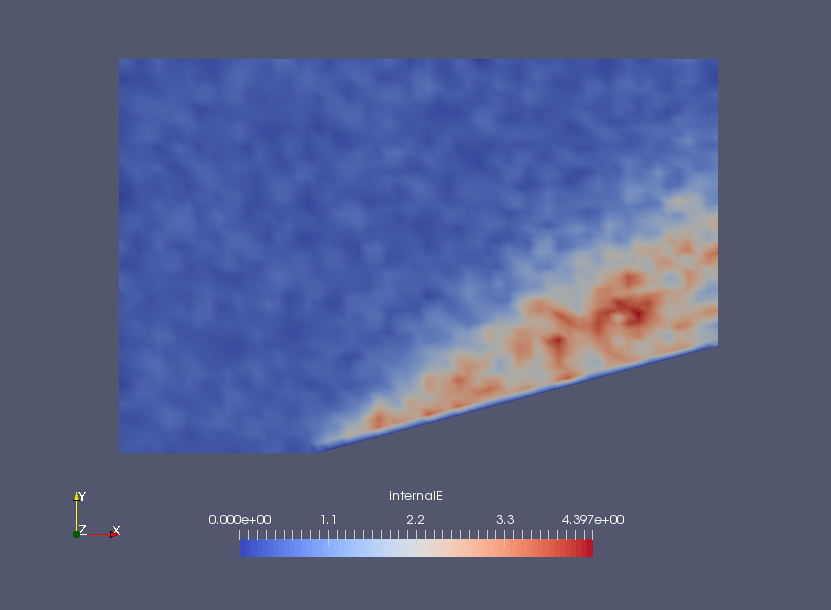﻿ Direct simulation Monte Carlo of supersonic flow hitting on a slope - XSim

# Direct simulation Monte Carlo of supersonic flow hitting on a slope

Update: January 3, 2018
OpenFOAM 4.x

## Case directory

\$FOAM_TUTORIALS/discreteMethods/dsmcFoam/wedge15Ma5

## Summary

We calculate the mixture gas flow of oxygen and nitrogen with the Direct Simulation Monte Carlo (DSMC) method. The Direct Simulation Monte Carlo (DSMC) method was proposed by G. A. Bird in the 1960s to calculate the flow of rarefied gases using the Boltzmann equation.

The properties of the gas are defined in the file "dsmcProperties" in the directory "constant", and the initial state is defined in the file "dsmcInitialiseDict" in the directory "system".

The model geometry is as follows: width 45.7 cm, height 30.0 cm, depth 0.84 cm. Among the boundaries, the region "flow" is assumed to be a flow velocity of (1736, 0, 0) m/s and the region "obstacle" is assumed to be a no-slip wall.Model geometry

We have configured the file "controlDict" in the directory "system" to use a function object to output the forces and moments acting on the region "obstacle" at each time. These values are output as a file "forces.dat" in the directory "postProcessing/forces1/0".

Each variable in directory 0 is as follows.

• boundaryT - Boundary temperature
• boundaryU - Boundary velocity
• dsmcRhoN - DSMC particles Density
• fD - Force Density (stress vector)
• q - Wall heat flux
• iDof - Density of internal degrees of freedom
• internalE - Internal energy density
• linearKE - Linear kinetic energy density
• momentum - Momentum density
• rhoM - Mass density
• rhoN - Number density
• dsmcSigmaTcRMax - maximum value of "sigmaT × cR" in each cell (see Bird p220)

Meshes are created before the calculation. The meshes are as follows.Meshes

The calculation result is as follows.Momentum densityInternal energy density

As explained earlier, the forces and moments acting on the region "obstacle" at each time are output to the file postProcessing/forces1/0/forces.dat. The forces and moments are output as pressure, viscous, and porous contribution (porous).

## Commands

cp -r \$FOAM_TUTORIALS/discreteMethods/dsmcFoam/wedge15Ma5 wedge15Ma5
cd wedge15Ma5

blockMesh
decomposePar
mpirun -np 4 dsmcInitialise -parallel
mpirun -np 4 dsmcFoam -parallel
reconstructPar -noLagrangian

paraFoam

When combining the divided data in each processors using the command reconstructPar, we added the option "-noLagrangian" to prevent unnecessary Lagrangian data from being combined.

## Calculation time

2 minutes 51.54 seconds *4 parallel, Inter(R) Core(TM) i7-2600 CPU @ 3.40GHz 3.40GHz